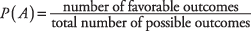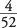## Classic Theory

The classic theory of probability underlies much of probability in statistics. Briefly, this theory states that the chance of a particular outcome occurring is determined by the ratio of the number of favorable outcomes (or “successes”) to the total number of outcomes. Expressed as a formula,For example, the probability of randomly drawing an ace from a well‐shuffled deck of cards is equal to the ratio. Four is the number of favorable outcomes (the number of aces in the deck), and 52 is the number of total outcomes (the number of cards in the deck). The probability of randomly selecting an ace in one draw from a deck of cards is, therefore,, or 0.077. In statistical analysis, probability is usually expressed as a decimal and ranges from a low of 0 (no chance) to a high of 1 (certainty).

The classic theory assumes that all outcomes have equal likelihood of occurring. In the example just cited, each card must have an equal chance of being chosen—no card is larger than any other or in any way more likely to be chosen than any other card.

The classic theory pertains only to outcomes that are mutually exclusive (or disjoint), which means that those outcomes may not occur at the same time. For example, one coin flip can result in a head or a tail, but one coin flip cannot result in a head and a tail. So, the outcome of a head and the outcome of a tail are said to be “mutually exclusive” in one coin flip, as is the outcome of an ace and a king in one card being drawn.